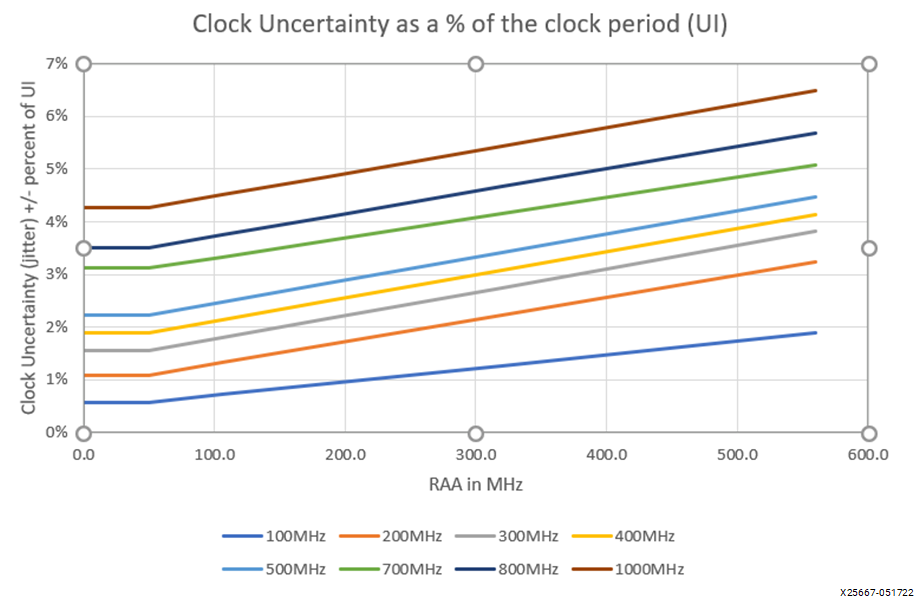# Methods to Reduce URAA

## Versal ACAP Clocking Resources Architecture Manual (AM003)

Document ID
AM003
Release Date
2022-05-24
Revision
1.4 English

The following sections explain how the pessimistic assumptions used in the URAA calculation in the previous section can be better estimated to reduce the final URAA value. For reference, the following chart shows the relationship between URAA and clock uncertainty.

Figure 1. Relationship between URAA and Clock Uncertainty## Finding a lower URAA using analysis of blocks of RAMs that are simultaneously enabled/disabled

If you can define the largest block of RAM that can be simultaneously enabled or disabled, then a lower URAA can be calculated. This calculation is done using the formula in the previous section. The sum of the RAM frequencies should be calculated using the largest block of RAM that will be simultaneously enabled or disabled. Simultaneously is defined as being enabled within 45 ns of each other. If a RAM instance can be determined to not switch within the window, it can be excluded. The information can be found from simulation but not static timing analysis.

For example, if a design uses 500 BRAM36 sites with an average frequency of 200 MHz and 300 URAM sites with an average frequency of 400 MHz, the RAA is calculated as follows:

Sum of the RAM frequencies = 0.5 * BRAM36_freq + URAM_freq

where,

BRAM36_freq = 500 BRAM36 sites * 200 MHz = 100,000 MHz
URAM_freq = 300 URAM sites * 400 MHz = 120,000 MHz
Sum of RAM frequencies = 0.5 * 100,000 MHz + 120,000 MHz = 170,000 MHz
Total number of RAMs = 946.5 (see Table 1)
URAA = 170,000 MHz / 946.5 = 179.6 MHz

Based on a knowledge of the design, only 250 BRAM36 sites and 100 URAM sites can be simultaneously enabled. In this case the calculation for RAA is reduced to:

Sum of the RAM frequencies = 0.5 * BRAM36_freq + URAM_freq

where,

BRAM36_freq = 250 BRAM36 sites * 200 MHz = 50,000 MHz
URAM_freq = 100 URAM sites * 400 MHz = 40,000 MHz
Sum of RAM frequencies = 0.5 * 50,000 MHz + 40,000 MHz = 65,000 MHz
Total number of RAMs = 946.5 (see Table 1)
URAA = 65,000 MHz / 946.5 = 68.7 MHz

In the previous example, if the group of 250 BRAM36 sites and the group of 100 URAM sites cannot be enabled together then the calculation must be done individually and the largest URAA value must be used.

Sum of the BRAM36 frequencies = 0.5 * BRAM36_freq

where,

BRAM36_freq = 250 BRAM36 sites * 200 MHz = 50,000 MHz
Sum of RAM frequencies = 0.5 * 50,000 MHz = 25,000 MHz
Total number of RAMs = 946.5 (see Table 1)
URAA(BRAM36) = 25,000 MHz / 946.5 = 26.4 MHz
Sum of the URAM frequencies = URAM_freq

where,

URAM_freq = 100 URAM sites * 400 MHz = 40,000 MHz
Sum of RAM frequencies = 40,000 MHz
Total number of RAMs = 946.5 (see Table 1)
URAA(URAM) = 40,000 MHz / 946.5 = 42.3 MHz
URAA = URAA(URAM) because URAA(URAM) > URAA(BRAM) = 42.3 MHz because 40.3 MHz 42.3 MHz > 26.4 MHz

For an example of reduction in the default clock uncertainty, refer to the section Specifying the RAM Activity for Jitter in Versal ACAP Hardware, IP, and Platform Development Methodology Guide (UG1387). The example provided in this section shows the difference in clock uncertainty (less pessimistic) of setting the USER_RAM_AVERAGE_ACTIVITY to 160 versus the pessimistic default of 480.

## Finding a lower URAA by limiting the rate at which RAMs can be enabled and disabled

If you can justify that the maximum rate for enabling and disabling the RAM is 22 MHz or less, then the URAA value can be reduced by a factor of 1.5.

A 22 MHz rate is equivalent to having 45 ns between enabling and disabling an individual RAM. For example, if a URAA is calculated at 300 MHz and the 22 MHz rule can be applied, then the URAA can be entered as 200 MHz (300 MHz/1.5). This factor would be applied after any calculations described earlier in this section.